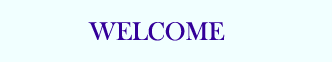TUTORIALS

## PHP Basic

PHP HOME
PHP Intro
PHP Install
PHP Syntax
PHP Variables
PHP String
PHP Operators
PHP If...Else
PHP Switch
PHP Arrays
PHP While Loops
PHP For Loops
PHP Functions
PHP Forms
PHP \$_GET
PHP \$_POST

PHP Date
PHP Include
PHP File
PHP Sessions
PHP E-mail
PHP Secure E-mail
PHP Error
PHP Exception
PHP Filter

## PHP Database

MySQL Introduction
MySQL Connect
MySQL Create
MySQL Insert
MySQL Select
MySQL Where
MySQL Order By
MySQL Update
MySQL Delete
PHP ODBC

XML Expat Parser
XML DOM
XML SimpleXML

AJAX Intro
AJAX PHP
AJAX Database
AJAX XML
AJAX Live Search
AJAX Poll

PHP Array
PHP Calendar
PHP Date
PHP Directory
PHP Error
PHP Filesystem
PHP Filter
PHP FTP
PHP HTTP
PHP Libxml
PHP Mail
PHP Math
PHP Misc
PHP MySQL
PHP SimpleXML
PHP String
PHP XML
PHP Zip

PHP Quiz
PHP Exam

# PHP Looping - For Loops

Loops execute a block of code a specified number of times, or while a specified condition is true.

## The for Loop

The for loop is used when you know in advance how many times the script should run.

### Syntax

 for (init; condition; increment)   {   code to be executed;   }

Parameters:

• init: Mostly used to set a counter (but can be any code to be executed once at the beginning of the loop)
• condition: Evaluated for each loop iteration. If it evaluates to TRUE, the loop continues. If it evaluates to FALSE, the loop ends.
• increment: Mostly used to increment a counter (but can be any code to be executed at the end of the loop)

Note: Each of the parameters above can be empty, or have multiple expressions (separated by commas).

### Example

The example below defines a loop that starts with i=1. The loop will continue to run as long as i is less than, or equal to 5. i will increase by 1 each time the loop runs:

 ";   } ?>

Output:

 The number is 1 The number is 2 The number is 3 The number is 4 The number is 5

## The foreach Loop

The foreach loop is used to loop through arrays.

### Syntax

 foreach (\$array as \$value)   {   code to be executed;   }

For every loop iteration, the value of the current array element is assigned to \$value (and the array pointer is moved by one) - so on the next loop iteration, you'll be looking at the next array value.

### Example

The following example demonstrates a loop that will print the values of the given array:

 ";   } ?>

Output:

 one two three